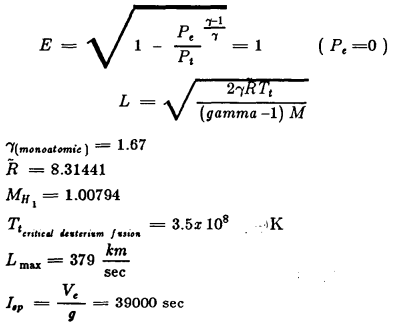## Friday, June 20, 2008Done! 74 pages of pure fun.

The formulae probably came out a bit weird so I'll have to change them if any real astrophysicists come along and complain, but for the time being it has all the data, and now it can be cut, copied, pasted, automatically translated into other languages and all the rest.

Next I'm going to put up the whole thing as a single post, and then turn it into a pdf and put it up on scribd.com as well.

 Probe wt.mt. No. SRB'sShuttleSize Delta Vkm/s S/C Total Wt.mt. 1409.695 .1 .205 1469.3 1409.695 .2 .401 1528.905 1409.695 .3 .589 1588.51 1409.695 .4 .768 1648.115 1409.695 .5 .94 1 707. 72 1409.695 .6 1.104 176 7.32.5 1409.695 .7 1.263 1826.93 1409.695 .8 1.415 1886.535 1409.695 .9 1.562 1946.14 1409.695 1 1.703 2005.745

Plane change of 9 degrees
Delta V required is 1.2123 km/s

 Probe wt.mt. No. SRB'sShuttleSize Delta Vkm/s S/C Total Wt.mt. 694.435 1 2.914 1280.485 694.435 1.1 3.112 1350.09 694.435 1.2 3.298 1409.695 694.435 1.3 3.475 1469.3 694.435 1.4 3.643 1528.905 694.435 1.5 3.802 1588.51

Escaping Earth
Delta V required is 3.2002 km/s

 Probe wt.mt. No. SRB'sShuttleSize Delta Vkm/s S/C Total Wt.mt. 396.41 .1 .689 456.015 396.41 .2 1.28 515.62 396.41 .3 1.795 575.225 396.41 .4 2.249 634.83 396.41 .5 2.653 694.435

Aiding in escaping solar system
Delta V required is at least 2.5 km/s

Laser-Pumped Light Sail

Assumptions:

1) 100% of laser is focused onto the sail continuously for one year.
2) the solar energy to pressure ratio holds true for the specific laser used.

At 1 A.U.:

1353 watts/square meter from the sun
and
4.6E-6 N/square meter from the sun

therefore,

2.94E8 watts/N.

Delta V required = 13500 km/sec
Time interval = 31,472,262 sec (1 year)

Therefore,

Required acceleration = .429 m/sec squared
and
The force required / 1268.8 N

So that with a laser operating at 100% efficiency

Required power = 3.78E12 watts

EXTREME HIGH TEMPERATURE EXPANSION OF GAS

"Ideal" Rocket Nozzle: Perfect Gas
Steady Flow - no shock, friction or heat losses
One Dimensional Flow
Frozen Chemical Equilibrium

Text:

Ve (exit velocity) = L E
L = Limiting Gas Velocity
E = Pressure Expansion RatioE = sqrt (1-Pe(gamma-1/gamma)/Pt) = 1 (Pe = 0)
L = sqrt (2 gamma RTt/gamma-1)M)
gamma (monoatomic) = 1.67
R = 8.31441
MH1 = 1.00794
Tt critical deuterium fusion = 3.5x 10^8 K
Lmax = 379 km/sec
Isp = Ve/g = 39000 sec

Fusion Reaction

He3 + He2 ----> He4 + H1

at reaction temperature:
He4 = d2+ + 2e-
H1 = p+1 + e-

the resulting charged particles can be magnetically funneled and used to charge induction coils upon exiting.

Pellet Size vs. FrequencyText:

.085g
HIGH MASS FLOW
LOW MASS FLOW
.005 g
14-H2
250 H2

an engineering analysis must be done on the proposed engine to determine pellet size and pulse frequency.Text:
PROCESSING BLOCK DIAGRAM
IR IMAGER
VISUAL IMAGER
UV IMAGER
PARTICLE DETECTORS
ASTRONOMETRIC TELESCOPES
ATTITUDE DETERMINATION
ATTITUDE / POSITION PROCESSOR
INSTRUMENT CONTROLS
DATA EVALUATION
SPACECRAFT ATTITUDE CONTROL
MISSION PROFILE
COMMUNICATION PROCESSOR
MISSION OBJECTIVES
LASERS

Sizing of the Fuel Tanks

1. Tank Volume

The storage density for the fuel is .0708 tons per cubic meter. Therefore, the total volume needed is:

Vol = (264.276 mt)*(1/.0708 mt/m^3)
= 3732.71 m^3

The radius of the fuel tanks is set at 2.5 meters and the length is then computed.

length = (Vol/6)/(pi*r^2)
= (3732.71 m^3/6) / (pi*(2.5)^2)
= 31.68 meters

The total volume is divided by six, because six fuel tanks are used.

2. Tank Thickness

A. Interstellar cruise phase

Newton's equation, F=ma, is used to find the forces on the fuel tanks during flight. The maximum force will occur when the acceleration is a maximum. This is when the mass is a minimum for a constant thrust problem. In our case, the minimum mass is:

Mmin = 2 fuel tanks + 6 straps + center section + engine + payload
= 89.345 metric tons

Therefore,

Amax = Thrust/m
= 1.838e3 N / 89.345e3 kg
= .021 m/s^2

The maximum force possible on the tanks could then be:

Fmax = (mass of fuel in tanks) * a
= 44.05e3 kg * .021 m/s^2
= 924.97 N

A safety factor of 5 is now applied to find the design load.

Fdes = 5 * F
= 4.62 kN

Next, the tank area that the force is acting on is found.

Area = pi * r^2
= pi * 2.5^2
= 19.635 m^2

So, the pressure will be:

p=F/A
= 4.62 kN / 19.635 m^2
= 235.54 N/m^2

The thickness of the tanks is now determined.

t = (P * r) / (2 * yield stress)

If we assume that we will use A1 1100-0, the yield stress is 3.45e7 N/m^2. This gives a thickness of:

t = (235.54 N/m^2*2.5 m)/(2*3.45e7 N/m^2)
= 8.53e-6 m or .00853 mm

Therefore, the fuel tanks can be made from 2 mm A1 1100-0 sheets.

B. Orbital Maneuvers

Since the upper stages will require advanced upper stages, the forces on the spacecraft are not known. Through the use of staging, multiple burns per maneuver, and the use of a stronger aluminum alloy, the thickness of the fuel tanks can be kept at 2 mm.

Item --- Components --- Weight Metric Tons
• Engine
• Chamber 4.35
• Igniter 17.543
• Field Coil 10.245
• Total 32.138
• Fuel Total 264.276
• Structure
• Fuel Tanks 55.038
• Straps 9.151
• Center Section 1.737
• Other Structure 4.074
• Total 70.00